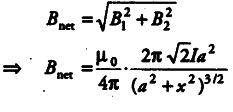# Two very small identical circular loops (1) and (2) carrying equal current

Two very small identical circular loops (1) and (2) carrying equal current I are placed vertically (with respect to the plane of the paper) with their geometrical axes perpendicular to each other as shown in the figure. Find the magnitude and direction of the net magnetic field produced at the point O.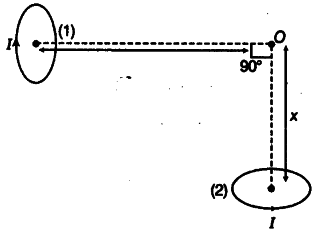The magnetic field at a point due to a circular loop is given by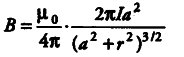where, / = current through the loop
a = radius of the loop
r = distance of O from the centre of the loop.
Since I, a and r = x are the same for both the loops, the magnitude of B will be the same and is given by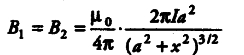The direction of magnetic field due to loop (1) will be away from 0 and that of the magnetic field due to loop (2) will be towards O as shown. The direction of the net magnetic field will be as shown below: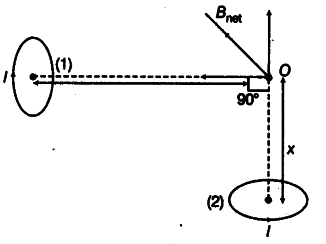The magnitude of the net magnetic field is given by